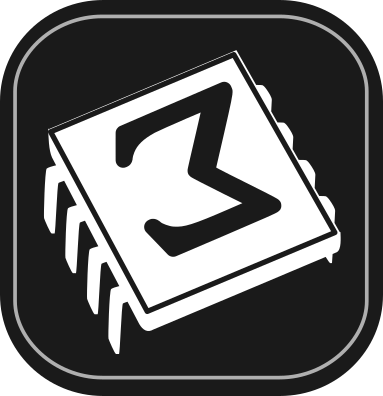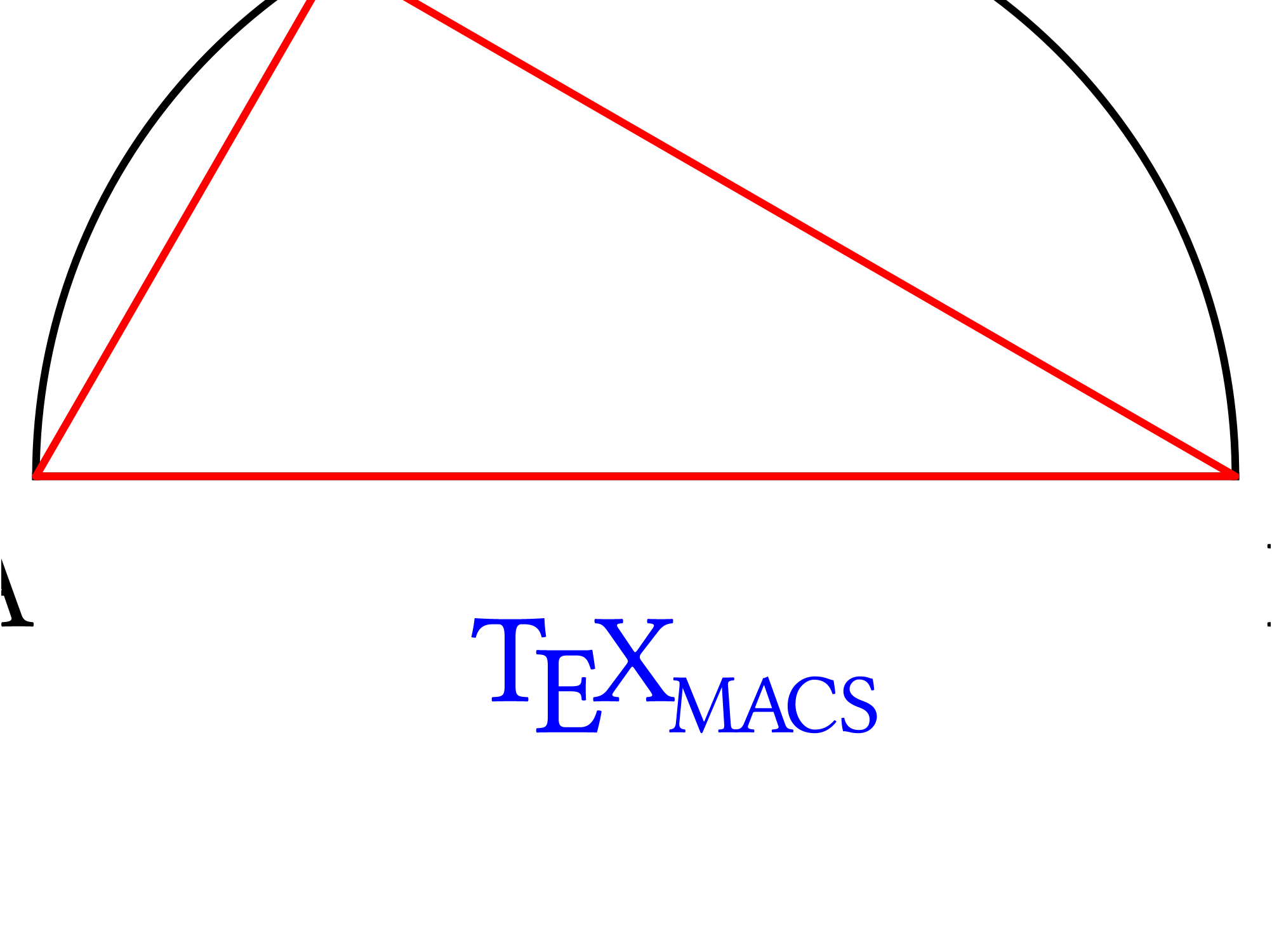[main]Notes on TeXmacs

# Embedding graphics composed with Scheme into documents

In a previous note, we have shown an example of TeXmacs native graphics generated with Scheme, a triangle inscribed in a half-circle.

Now let us see how to embed it seamlessly in a document. Our work is based on Fold Executable environments, available under InsertFoldExecutable (and then choose the Scheme option).

Since in each Executable environment it is possible to execute one Scheme instruction only, we will wrap all of the code inside a begin form.

For more complex drawings users may feel the need of more efficient facilities to compose, test and deploy into TeXmacs their code. We are going to discuss some available tools in a future post.

A synthetic discussion of TeXmacs graphics primitives and how to code them in Scheme is in the previous note (see also the manual, in HelpManualCreating technical pictures). Here let us just remind that TeXmacs graphics are composed by listing graphical objects, each made by the application of a graphical primitive, inside a graphics primitive.

In the code we ue in this note, we introduce a function pt that generates point primitives, (which are parametrized by two numbers, expressed as strings), and with it we generate points that we will use to build other primitives (arc, line, cline and text-at).

In turn, TeXmacs will use the primitives to represent the triangle, the half-circle and the decorations, that is the drawing that we will embed in the document.

We open the environment with InsertFoldExecutableScheme and we obtain the yellow box we got used to in the note on embedding TikZ graphics, in this case introduced by a Scheme title on a gray background:

 Scheme

Press ⇧Return and type or paste the graphics command; in this note let us copy the same commands that generate the drawing in the previous note, wrapping everything in a begin form as we already said:

 Scheme ```(begin (define pi (acos -1)) ;; a function for generating TeXmacs points (define (pt x y) ‘(point ,(number->string x) ,(number->string y))) ;; points for the triangle (define pA (pt -2 0)) (define pB (pt 2 0)) (define xC (- (* 2 (cos (/ pi 3))))); x-coordinate for point C (define yC (* 2 (sin (/ pi 3)))); y-coordinate for point C (define pC (pt xC yC)) ;; points for the letters (define tA (pt -2.3 -0.5)) (define tB (pt 2.1 -0.5)) (define tC (pt (- xC 0.2) (+ yC 0.2))) (stree->tree ‘(with "gr-geometry" (tuple "geometry" "400px" "300px" "center") (graphics ;; the arc and the line together make the semicircle (with "color" "black" (arc ,pA ,pC ,pB)) (with "color" "black" (line ,pA ,pB)) ;; a closed polyline for the triangle (with "color" "red" (cline ,pA ,pB ,pC)) ;; add letters using text-at (with "color" "black" (text-at "A" ,tA)) (with "color" "black" (text-at "B" ,tB)) (with "color" "black" (text-at "C" ,tC)) ;; finally decorate with the TeXmacs symbol ;; and close all of the parentheses!!! (with "color" "blue" (text-at (TeXmacs) ,(pt -0.55 -0.75)))))))```

The last step is pressing ⇧Return to execute the code and generate the drawing. We do it in a big-figure environment (InsertImageBig figure) to demonstrate seamless embedding:Figure 1. A drawing generated with Scheme, embedded in a big-figure environment

The drawing we generated is editable in two different ways.

Placing the cursor at the drawing (just after or just before, the drawing is then surrounded by a thin cyan frame) and pressing Return brings back the yellow edit window, where the code can be changed and re-executed into a new drawing.

 Scheme ```(begin (define pi (acos -1)) ;; a function for generating TeXmacs points (define (pt x y) ‘(point ,(number->string x) ,(number->string y))) ;; points for the triangle (define pA (pt -2 0)) (define pB (pt 2 0)) (define xC (- (* 2 (cos (/ pi 3))))); x-coordinate for point C (define yC (* 2 (sin (/ pi 3)))); y-coordinate for point C (define pC (pt xC yC)) ;; points for the letters (define tA (pt -2.3 -0.5)) (define tB (pt 2.1 -0.5)) (define tC (pt (- xC 0.2) (+ yC 0.2))) (stree->tree ‘(with "gr-geometry" (tuple "geometry" "400px" "300px" "center") (graphics ;; the arc and the line together make the semicircle (with "color" "black" (arc ,pA ,pC ,pB)) (with "color" "black" (line ,pA ,pB)) ;; a closed polyline for the triangle (with "color" "red" (cline ,pA ,pB ,pC)) ;; add letters using text-at (with "color" "black" (text-at "A" ,tA)) (with "color" "black" (text-at "B" ,tB)) (with "color" "black" (text-at "C" ,tC)) ;; finally decorate with the TeXmacs symbol ;; and close all of the parentheses!!! (with "color" "blue" (text-at (TeXmacs) ,(pt -0.55 -0.75)))))))```

It is also possible to edit the drawing with the interactive (point and click) facilities. In this case too it is always possible to return to the text-editing mode of the Executable environment by pressing Return with the cursor at the drawing, but if one does that, the interactive modifications are lost, i.e. one gets back to the Scheme code one had typed into the Executable environment.